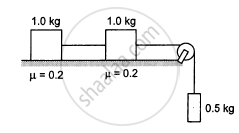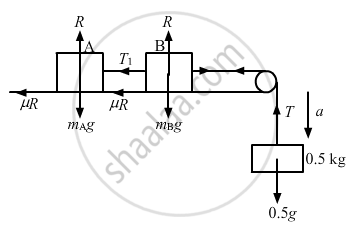Department of Pre-University Education, KarnatakaPUC Karnataka Science Class 11
Advertisement Remove all ads

# Consider the Situation Shown in the Following Figure. Calculate (A) the Acceleration of the - Physics

Sum

Consider the situation shown in the following figure. Calculate (a) the acceleration of the 1.0 kg blocks, (b) the tension in the string connecting the 1.0 kg blocks and (c) the tension in the string attached to 0.50 kg.Advertisement Remove all ads

#### SolutionFrom the above diagrams:
T + ma − mg = 0
T + 0.5a − 0.5 g = 0                       (1)
μR + ma + T1 − T = 0
μR + 1a + T1 − T = 0                      (2)
μR + 1a − T1 = 0
μR + a = T1                                    (3)
From Equations (2) and (3) we have
μR + a = T − T1
⇒ T − T1 = T1
⇒ T = 2T1
So, Equation (2) becomes
μR + a + T1 − 2T1 = 0
⇒ μR + a − T1 = 0
⇒ T1 = μR + a
= 0.2g + a                       (4)

and Equation (1) becomes
2T1 + 0.5a − 0.5g = 0
=> T_1=(0.5g-0.5a)/2
= 0.25g - 0.25a                    (5)
From Equations (4) and (5)
0.2g + a = 0.25g − 0.25a
=>a=0.05/1.25xx10
= 0.4 x 10 m/s2                     [g = 10 m/s2]
Therefore,
(a) the acceleration of each 1 kg block is 0.4 m/s2,
(b) the tension in the string connecting the 1 kg blocks is
T1 = 0.2g + a + 0.4 = 2.4 N
​    and
(c) the tension in the string attached to the 0.5 kg block is
T = 0.5g − 0.5a
= 0.5 × 10 − 0.5 × 0.4
= 4.8 N.

Is there an error in this question or solution?
Advertisement Remove all ads

#### APPEARS IN

HC Verma Class 11, 12 Concepts of Physics 1
Chapter 6 Friction
Exercise | Q 11 | Page 97
Advertisement Remove all ads
Advertisement Remove all ads
Share
Notifications

View all notifications

Forgot password?